# Constructing and fitting admixture graphs to D statistics

#### 2016-12-13

This document describes the admixturegraph package, a package for fitting admixture graphs to genetic data.

The package enables you to:

• specify and visualize admixture graphs
• extract the implied equations a given graph makes for predicting correlations of genetic drift as specified by the expected $$f_2$$, $$f_3$$ and $$f_4$$ statistics
• fit a graph to data summarized as $$D$$ statistics and test the goodness of fit
• explore the graph space over a given set of populations with combination of brute force and heuristics

# Admixture graphs and genetic drift

Gene frequencies in related populations are correlated. If two populations split apart a certain time in the past, all gene frequencies were the same at that split time — where the populations were one and the same — and has since drifted apart in the time since — where the populations have evolved independently.

With three related populations where one branched off a common ancestor early and the other two later in time the two closest related populations will, all else being equal, have more correlated gene frequencies than they have with the third population.

All else being equal here means that the populations should have evolved at roughly the same speed — the gene frequency changes should not be more dramatic in one population than another. If we consider populations A, B and C, with A and B closest relatives but where population A has changed very rapidly since it split from population B, then gene frequencies in A and B could be less correlated than between B and C (but A and C would be even less correlated).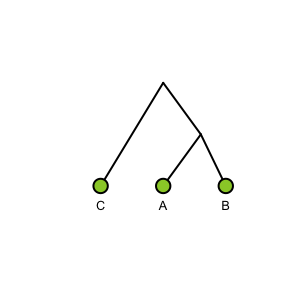The way gene frequencies change over time as a population evolve is called genetic drift and the way gene frequencies are correlated between populations is determined by how they are related. These relationships can be simple trees with ancestral populations split into descendant populations or more complex graphs with gene flow between diverged populations.

Assuming that populations evolve by either splitting into descendant populations or merging in admixture events — but do not experience periods of continuous gene flow — their relationship can be described as a so-called admixture graph (Patterson et al. 2012). Such a graph not only describes the history of the populations but can also be seen as a specification of how gene frequencies in the populations will be correlated. Different summary statistics can capture the correlations in gene frequencies between populations, extracting different aspects of this correlation, and the expected values of these statistics can be computed as polynomials over branch lengths and admixture proportions in the admixture graph.

# Building and visualizing admixture graphs

In the admixturegraph package, graphs are built by specifying the edges in the graph and naming the admixture proportions so these have a variable associated.

It is first necessary to specify the nodes in the graphs, where there is a distinction between leaves and inner nodes so leaves later can correspond to values in $$f$$ statistics computed from genetic data. It is necessary to name the nodes so we have something to refer to when specifying the edges.

Edges are specified by specifying the parent of each node. Only one node is allowed not to have a parent; the package cannot deal with more than one root.

The tree shown above is specified as:

leaves <- c("A", "B", "C")
inner_nodes <- c("AB", "ABC")
edges <- parent_edges(c(edge("A", "AB"),
edge("B", "AB"),
edge("AB", "ABC"),
edge("C", "ABC")))
graph <- agraph(leaves, inner_nodes, edges)

and plotted like:

plot(graph)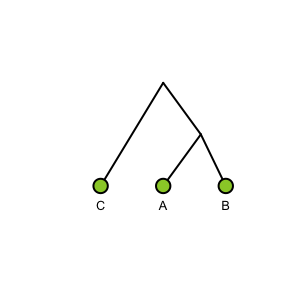By default the labels on inner nodes are not shown but this can be changed by:

plot(graph, show_inner_node_labels = TRUE)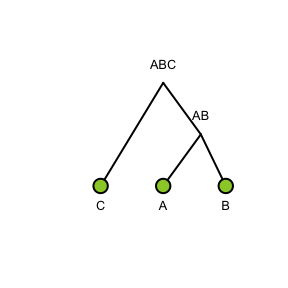The shape of the output of the plotting algorithm is determined by heuristics designed to make the graph visually pleasing. However, the user has the final say on the shape if what the heuristics propose is not satisfactory (like the non-alphabetical order of leaves in the example above).

Admixture events are specified by having admixture edges. These are edges with two parents. Admixture edges really represent two different edges, capturing the drift between the two populations ancestral to the admixture event before the admixture took place, so we typically have to add inner nodes on the edges where these two ancestral populations branched off other lineages in the graph.

leaves <- c("A", "B", "C")
inner_nodes <- c("a", "c", "ABC")
edges <- parent_edges(c(edge("A", "a"), edge("a", "ABC"),
edge("C", "c"), edge("c", "ABC"),
admixture_edge("B", "a", "c", "alpha")))
graph <- agraph(leaves, inner_nodes, edges)

plot(graph, show_inner_node_labels = TRUE)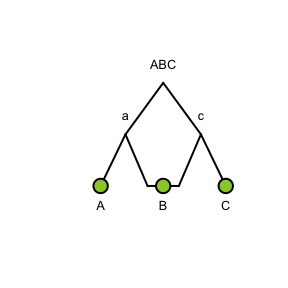Since we usually model admixtures that happened at some point in the past we usually also need an inner node above the present day admixed population.

leaves <- c("A", "B", "C")
inner_nodes <- c("a", "c", "b", "ABC")
edges <- parent_edges(c(edge("A", "a"), edge("a", "ABC"),
edge("C", "c"), edge("c", "ABC"),
edge("B", "b"),
admixture_edge("b", "a", "c", "alpha")))
graph <- agraph(leaves, inner_nodes, edges)

plot(graph, show_inner_node_labels = TRUE)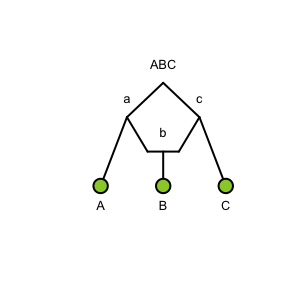This graph doesn’t contain a variable for the admixture proportions between the two ancestral populations to B. To work with the equations for expected drift statistics we need to specify this as well.

This is done through the last parameter when constructing the admixture graph, like this:

leaves <- c("A", "B", "C")
inner_nodes <- c("a", "c", "b", "ABC")
edges <- parent_edges(c(edge("A", "a"), edge("a", "ABC"),
edge("C", "c"), edge("c", "ABC"),
edge("B", "b"),
admixture_edge("b", "a", "c", "alpha")))
graph <- agraph(leaves, inner_nodes, edges)

plot(graph, show_inner_node_labels = TRUE, show_admixture_labels = TRUE)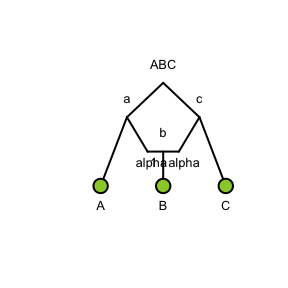As a somewhat more complex example we build a graph for samples of polar and brown bears described in (Cahill et al. 2015). Although the brown bears from mainland Alaska and even from Sweden were found to have some polar bear ancestry, the most remarkable episode of polar bear gene flow was into the population of brown bears that colonized Admiralty, Baranof and Chichagof islands of Alaska (the ABC bears).

A graph constructed to capture this main gene flow could look like this (the parameter platform is to make the horizontal lines around the admixture node a bit wider to make room for the labels, for more options on customising the graph plots see the documentation of plot.agraph):

leaves <- c("BLK", "PB", "Adm1", "Adm2", "Bar", "Chi1", "Chi2", "Denali", "Kenai", "Sweden")
inner_nodes <- c("R", "r", "s", "t", "u", "v", "w", "x", "y", "z", "M")
edges <- parent_edges(c(edge("BLK", "R"),
edge("PB", "s"),
edge("Adm1", "x"),
edge("Adm2", "x"),
edge("Bar", "y"),
edge("Chi1", "z"),
edge("Chi2", "z"),
edge("Denali", "v"),
edge("Kenai", "u"),
edge("Sweden", "t"),
edge("r", "R"),
edge("s", "r"),
edge("t", "r"),
edge("u", "t"),
edge("v", "u"),
edge("w", "M"),
edge("x", "w"),
edge("y", "w"),
edge("z", "y"),
admixture_edge("M", "s", "v", "a")))
bears_graph <- agraph(leaves, inner_nodes, edges)

plot(bears_graph, platform = 1.4, show_admixture_labels = TRUE)
#> fminbnd:  Exiting: Maximum number of function evaluations has been exceeded
#>          - increase MaxFunEvals option.
#>          Current function value: 2977.35463730617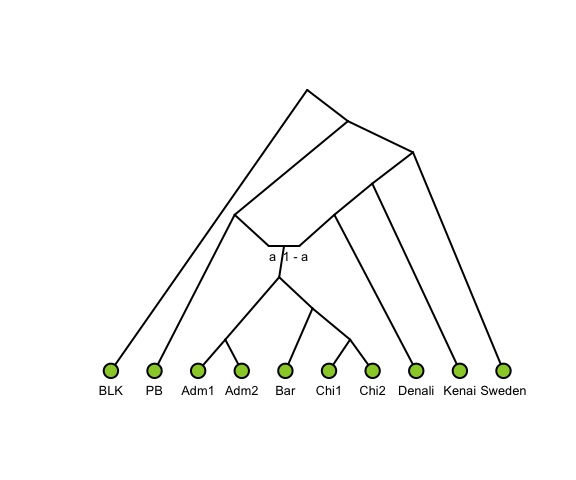# Extracting drift equations for expected $$f$$ statistics

The expectation of $$f_2$$, $$f_3$$, and $$f_4$$ statistics can be expressed in terms of admixture proportions and edge lengths of an admixture graph (Patterson et al. 2012). In the admixturegraph package these can be extracted from a graph using the functions sf2, sf3 and sf4 (where the “s” stands for “symbolic”).

The expected values are computed from weighted overlapping paths between pairs of edges such that $$f_4(A,B;C,D)$$ is the sum of overlapping paths from $$A$$ to $$B$$ with paths from $$C$$ to $$D$$, weighted by the probability of lineages taking each path. The $$f_2$$ and $$f_3$$ statistics can be seen as special cases of $$f_4$$ statistics: $$f_3(A;B,C)=f_4(A,B;A,C)$$ and $$f_2(A,B)=f_4(A,B;A,B)$$.

The expressions extracted using the sf2, sf3, and sf4 functions are in the form of (non-simplified) R expressions.

sf2(bears_graph, "Bar", "Chi1")
#> expression(edge_y_Bar + edge_y_z + edge_z_Chi1)
sf3(bears_graph, "Bar", "Chi1", "Chi2")
#> expression(edge_y_Bar + edge_y_z)
sf4(bears_graph, "BLK", "Chi1", "Bar", "Chi2")
#> expression(a * (edge_y_z) + (1 - a) * (edge_y_z))

# Fitting admixture graphs to observed $$f$$ statistics

Given a data set summarized as $$f$$ (or $$D$$) statistics admixturegraph can fit the edge lengths and admixture proportions of a graph to the data.

For fitting data the package expects the observed statistics in a data frame with at least the following columns: W, X, Y, and Z determining the statistic $$f_4(W,X,Y,Z)$$ and D, the actual value of the statistic (called $$D$$ since that is the header used if these are computed with Patterson et al.s ADMIXTOOLS package). Optionally, the data frame might also contain Z.values, the statistics divided by the standard error.

For the bear samples we have the following statistics (Cahill et al. 2015):

bears
#>      W  X      Y      Z      D Z.value
#> 1  BLK PB Sweden   Adm1 0.1258    12.8
#> 2  BLK PB  Kenai   Adm1 0.0685     5.9
#> 3  BLK PB Denali   Adm1 0.0160     1.3
#> 4  BLK PB Sweden   Adm2 0.1231    12.2
#> 5  BLK PB  Kenai   Adm2 0.0669     6.1
#> 6  BLK PB Denali   Adm2 0.0139     1.1
#> 7  BLK PB Sweden    Bar 0.1613    14.7
#> 8  BLK PB  Kenai    Bar 0.1091     8.9
#> 9  BLK PB Denali    Bar 0.0573     4.3
#> 10 BLK PB Sweden   Chi1 0.1786    17.7
#> 11 BLK PB  Kenai   Chi1 0.1278    11.3
#> 12 BLK PB Denali   Chi1 0.0777     6.4
#> 13 BLK PB Sweden   Chi2 0.1819    18.3
#> 14 BLK PB  Kenai   Chi2 0.1323    12.1
#> 15 BLK PB Denali   Chi2 0.0819     6.7
#> 16 BLK PB Sweden Denali 0.1267    14.3
#> 17 BLK PB  Kenai Denali 0.0571     5.6
#> 18 BLK PB Sweden  Kenai 0.0719     9.6

Since we have the Z.values we may visualize the statistics together with their confidence intervals (now the observations are considered as samples from a multivariate normal distribution):

plot(f4stats(bears))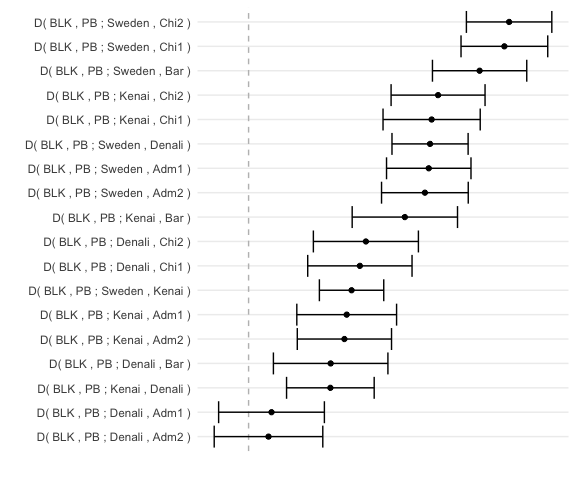Fitting the graph above to this data is done either with fit_graph (lots of details about the fit) or fast_fit (only the essentials but a bit faster, designed for usage in big loops).

Both functions use a combination of linear algebra and numerical optimisation (Nelder-Mead) to find the edge lengths and admixture proportions that minimize the value of $$(F-f)^t*S^{-1}*(F-f)$$. Here $$F$$ and $$f$$ are the vectors of predicted (from the graph with sf4 etc.) and observed (D from the data frame) statistics, respectively, and $$S$$ is the covariance matrix of the observed statistics $$f$$, either given by the user or replaced by a default proxy of the identity matrix or a diagonal matrix constructed from the Z.values. This cost function $$(F-f)^t*S^{-1}*(F-f)$$ can be interpreted as the log likelihood of the graph parameters (edge lengths and admixture proportions), up to an affine normalization. See the documentation of cost_function, edge_optimisation_function and log_likelihood for more info.

bears_fit <- fit_graph(bears, bears_graph)
#> fminbnd:  Exiting: No possible improvement of cost function.

The essentials about the fit can be printed with print.agraph_fit and visualized with plot.agraph_fit:

summary(bears_fit)
#>
#> Call: inner_fit_graph(data, graph, point, Z.value, concentration, optimisation_options,
#>     parameters, iteration_multiplier, qr_tol)
#>
#> None of the proportions {a} affect the quality of the fit!
#>
#> Optimal admixture proportions:
#>   a
#> 0.5
#>
#> Optimal edge lengths:
#>    edge_R_BLK      edge_R_r      edge_r_s      edge_r_t     edge_s_PB
#>     0.0000000     0.0000000     0.2174268     0.0000000     0.0000000
#>      edge_s_M edge_t_Sweden      edge_t_u  edge_u_Kenai      edge_u_v
#>     0.0000000     0.0000000     0.0000000     0.0000000     0.0000000
#> edge_v_Denali      edge_v_M      edge_w_x      edge_w_y   edge_x_Adm1
#>     0.0000000     0.0000000     0.0000000     0.0000000     0.0000000
#>   edge_x_Adm2    edge_y_Bar      edge_y_z   edge_z_Chi1   edge_z_Chi2
#>     0.0000000     0.0000000     0.0000000     0.0000000     0.0000000
#>      edge_M_w
#>     0.0000000
#>
#> Solution to a homogeneous system of edge lengths with the optimal admixture proportions:
#> Adding any such solution to the optimal one will not affect the error.
#>
#> Free edge lengths:
#> edge_R_BLK
#> edge_R_r
#> edge_r_t
#> edge_s_PB
#> edge_s_M
#> edge_t_Sweden
#> edge_t_u
#> edge_u_Kenai
#> edge_u_v
#> edge_v_Denali
#> edge_v_M
#> edge_w_x
#> edge_w_y
#> edge_x_Adm1
#> edge_x_Adm2
#> edge_y_Bar
#> edge_y_z
#> edge_z_Chi1
#> edge_z_Chi2
#> edge_M_w
#>
#> Bounded edge lengths:
#> edge_r_s = 0
#>
#> Minimal error:
#> 631.518
plot(bears_fit)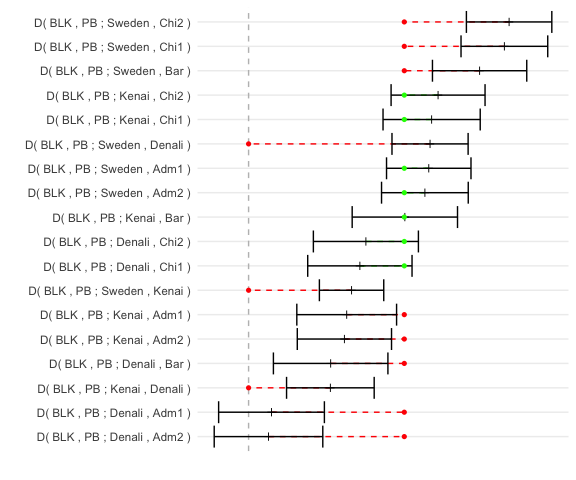It turned out that the value of the admixture proportion a had no effect on the minimum of the cost function. This is because our data frame wasn’t sufficient for a graph this big, which is also reflected by the fact that only one of the edge lengths (between polar bears and brown bears splitting and polar bears and the population mixing into ABC bears splitting) matter while the rest can be chosen freely. From the plot it is clear that this graph is not very convincing match with the data, and that additional gene flow from the polar bears into mainland Alaska bears would help.

With sufficient data the effect of admixture proportions on the cost function can be examined with the help of the functions one_dimensional_plot and contour_plot. See also coef.agraph_fit, fitted.agraph_fit, print.agraph_fit and residuals.agraph_fit.

# Exploring the graph space

The number of different admixture graphs grows extremely fast with the number of leaves and admixture events, so determining the phylogeny of a given set of populations by fitting every graph to the data with brute force might not be feasible. We provide some tools for restricting the search space.

For just a few populations and a few admixture events admixturegraph contains the complete collections of possible graphs. The graphs are stored as logical matrices in the data files graphs_x_y.RData, where x is the number of leaves and y the number of admixture events. Each row in such a matrix corresponds to a unique graph with the first capital letters of the alphabet as the population names; use the function vector_to_graph to interpret the vector as a graph and rename_nodes to rename the nodes.

example_graph <- vector_to_graph(graphs_3_1[1, ])
new_names <- list(A = "P1", B = "P2", C = "P3")
example_graph <- rename_nodes(example_graph, new_names)
plot(example_graph)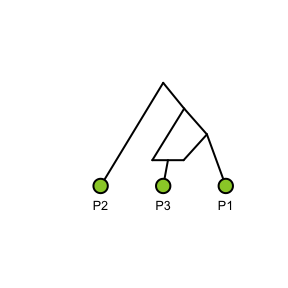A given graph can be expanded to a list of bigger graphs by several functions. To add a new leaf to an existing graph use add_a_leaf:

example_list_1 <- add_a_leaf(example_graph, "P4")

original <- graphics::par()$mfrow graphics::par(mfrow = c(3, 2)) for (graph in example_list_1) { plot(graph) }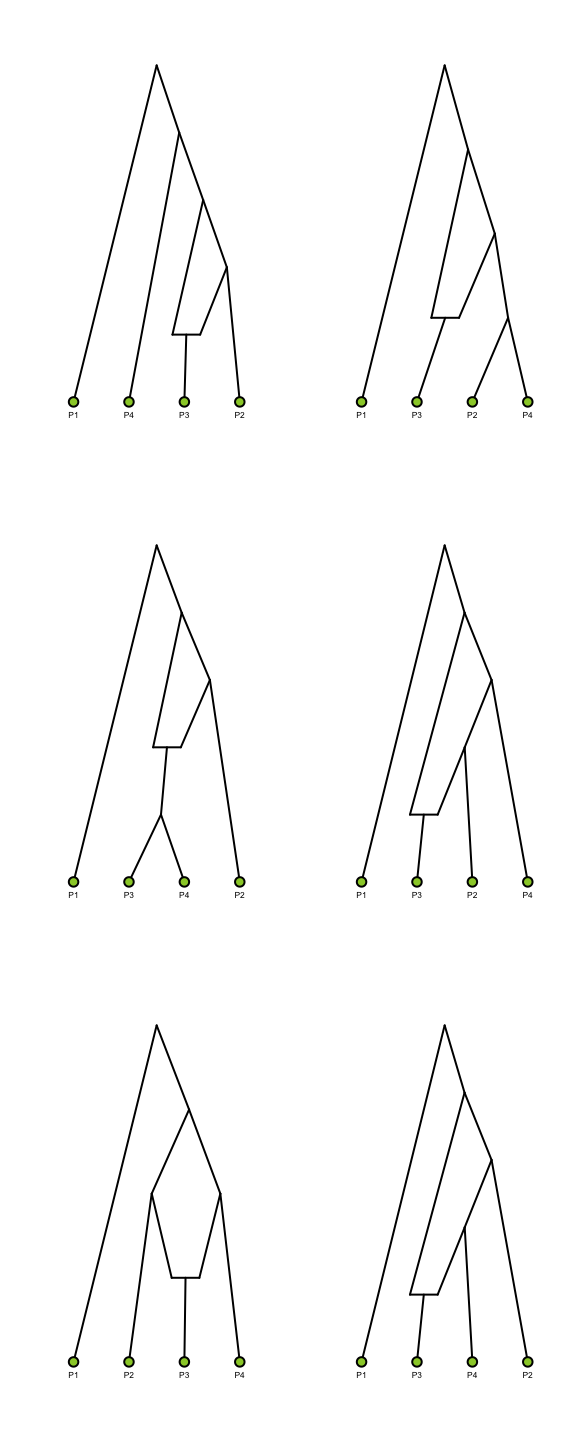graphics::par(mfrow = original) To add a new admixture event to an existing graph use either add_an_admixture or add_an_admixture2; the former is only adding one parental edge assuming the other one already exist in the graph and the latter is detaching one edge and reattaching it with two parental edges anywhere in the original graph (provided that the result still is a valid admixture graph): example_list_2 <- add_an_admixture(example_graph, "p2") length(example_list_2) #>  15 example_list_3 <- add_an_admixture2(example_graph, "p2") length(example_list_3) #>  33 plot(example_list_2[])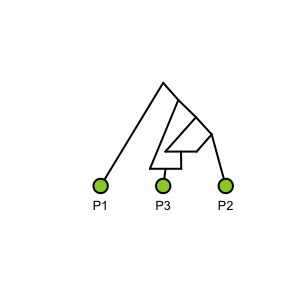None of these functions care where the original root was — its position has no effect on the predictions sf2, sf3 and sf4 as long as the admixture events remain the same. However, if we want we can select any non-admixed leaf as an outgroup, meaning that the root will be placed on the edge leading to the leaf: graph_with_a_new_root <- make_an_outgroup(example_list_2[], "P2") plot(graph_with_a_new_root)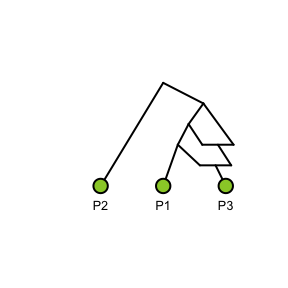# Model comparisons and parameter posteriors The package provides an MCMC for sampling over posterior probabilities of parameters and, by integrating out parameters, for computing the likelihood of a topology which in turn can be used to compute Bayes factors for comparing two topologies. To illustrate the use of this MCMC we consider two alternative topologies for the bears data contained in the package, one that models polar bears as admixed: leaves <- c("BLK", "PB", "Bar", "Chi1", "Chi2", "Adm1", "Adm2", "Denali", "Kenai", "Sweden") inner_nodes <- c("R", "X", "Z", "A", "B", "C", "D", "E", "F", "G", "H") edges <- parent_edges(c(edge("BLK", "R"), edge("PB", "Z"), admixture_edge("Z", "X", "E", "a"), edge("X", "R"), edge("Chi1", "G"), edge("Chi2", "G"), edge("Bar", "F"), edge("G", "F"), edge("F", "E"), edge("E", "D"), edge("Adm1", "H"), edge("Adm2", "H"), edge("H", "D"), edge("D", "C"), edge("Denali", "C"), edge("C", "B"), edge("Kenai", "B"), edge("B", "A"), edge("Sweden", "A"), edge("A", "X"))) bears_graph_1 <- agraph(leaves, inner_nodes, edges) plot(bears_graph_1) #> fminbnd: Exiting: Maximum number of function evaluations has been exceeded #> - increase MaxFunEvals option. #> Current function value: 3501.92808122963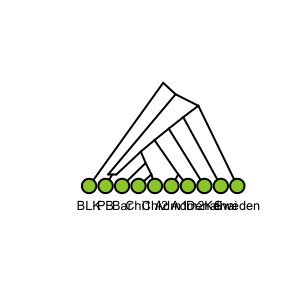and another that considers brown bears admixed, but with several waves of admixture for capturing that the f4 statistics indicate that some brown bears are closer related to polar bears than others. leaves <- c("BLK", "PB", "Bar", "Chi1", "Chi2", "Adm1", "Adm2", "Denali", "Kenai", "Sweden") inner_nodes <- c("R", "PBBB", "Adm", "Chi", "BC", "ABC", "x", "y", "z", "pb_a1", "pb_a2", "pb_a3", "pb_a4", "bc_a1", "abc_a2", "x_a3", "y_a4") edges <- parent_edges(c(edge("BLK", "R"), edge("PB", "pb_a1"), edge("pb_a1", "pb_a2"), edge("pb_a2", "pb_a3"), edge("pb_a3", "pb_a4"), edge("pb_a4", "PBBB"), edge("Chi1", "Chi"), edge("Chi2", "Chi"), edge("Chi", "BC"), edge("Bar", "BC"), edge("BC", "bc_a1"), edge("Adm1", "Adm"), edge("Adm2", "Adm"), admixture_edge("bc_a1", "pb_a1", "ABC", "a"), edge("Adm", "ABC"), edge("ABC", "abc_a2"), admixture_edge("abc_a2", "pb_a2", "x", "b"), edge("Denali", "x"), edge("x", "x_a3"), admixture_edge("x_a3", "pb_a3", "y", "c"), edge("Kenai", "y"), edge("y", "y_a4"), admixture_edge("y_a4", "pb_a4", "z", "d"), edge("Sweden", "z"), edge("z", "PBBB"), edge("PBBB", "R"))) bears_graph_2 <- agraph(leaves, inner_nodes, edges) plot(bears_graph_2) #> fminbnd: Exiting: Maximum number of function evaluations has been exceeded #> - increase MaxFunEvals option. #> Current function value: 3029.65995636151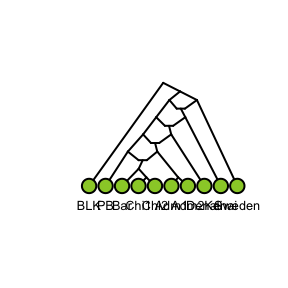Both graphs provide a reasonably good fit to the data, but the second is of course more complex than the first. plot(fit_graph(bears, bears_graph_1)) #> fminbnd: Exiting: No possible improvement of cost function.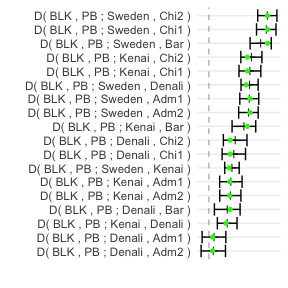plot(fit_graph(bears, bears_graph_2))To use the MCMC we first need to extract relevant information from the data and the graphs to build the Markov chain. This is done using the make_mcmc_model function. mcmc1 <- make_mcmc_model(bears_graph_1, bears) mcmc2 <- make_mcmc_model(bears_graph_2, bears) When constructing the MCMC, the package works out which parameters can be inferred from the avialable $$f$$-statistics. With this toy example there is not information about any of the terminal edges, since $$f_4$$ statistics do not provide information about this, so the parameters we can sample over are fewer than the graphs actually contain. mcmc1$parameter_names
#>  "a"        "edge_A_B" "edge_B_C" "edge_C_D" "edge_D_E"
mcmc2$parameter_names #>  "a" "b" "c" #>  "d" "edge_PBBB_pb_a4" "edge_pb_a2_pb_a1" #>  "edge_pb_a3_pb_a2" "edge_pb_a4_pb_a3" To sample from the MCMCs we use the function run_metropolis_hasting. It requires an initial state for the Markov chain. With MCMCs it is usually a good idea to sample several chains with different initial states, but for the purpose of this document we just use a single starting point for each model, and simply use 0.5 for all parameters. initial1 <- rep(0.5, length(mcmc1$parameter_names))
initial2 <- rep(0.5, length(mcmc2\$parameter_names))

chain1 <- run_metropolis_hasting(mcmc1, initial1, iterations = 10000, verbose = FALSE)
chain2 <- run_metropolis_hasting(mcmc2, initial2, iterations = 10000, verbose = FALSE)

Once the chains have run, we can extract posterior samples, corresponding likelihoods, and posterior distributions from the chains. These chains can be analysed using various summary statistics such as implemented in the coda package, but for the purpose of this documentation we just show the trace of one parameter for each of the chains:

head(chain1)
#>        a edge_A_B edge_B_C edge_C_D edge_D_E     prior likelihood
#> [1,] 0.5      0.5      0.5      0.5      0.5 -5.555599   -22960.9
#> [2,] 0.5      0.5      0.5      0.5      0.5 -5.555599   -22960.9
#> [3,] 0.5      0.5      0.5      0.5      0.5 -5.555599   -22960.9
#> [4,] 0.5      0.5      0.5      0.5      0.5 -5.555599   -22960.9
#> [5,] 0.5      0.5      0.5      0.5      0.5 -5.555599   -22960.9
#> [6,] 0.5      0.5      0.5      0.5      0.5 -5.555599   -22960.9
#>      posterior
#> [1,] -22966.45
#> [2,] -22966.45
#> [3,] -22966.45
#> [4,] -22966.45
#> [5,] -22966.45
#> [6,] -22966.45
plot(chain1[, "a"])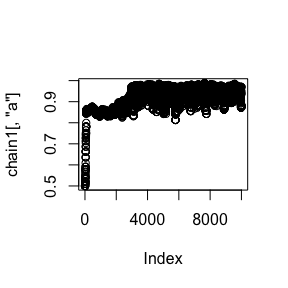head(chain2)
#>              a         b         c         d edge_PBBB_pb_a4
#> [1,] 0.5000000 0.5000000 0.5000000 0.5000000       0.5000000
#> [2,] 0.5000000 0.5000000 0.5000000 0.5000000       0.5000000
#> [3,] 0.5000000 0.5000000 0.5000000 0.5000000       0.5000000
#> [4,] 0.5000000 0.5000000 0.5000000 0.5000000       0.5000000
#> [5,] 0.4807834 0.5113388 0.5079567 0.4881515       0.5082393
#> [6,] 0.4807834 0.5113388 0.5079567 0.4881515       0.5082393
#>      edge_pb_a2_pb_a1 edge_pb_a3_pb_a2 edge_pb_a4_pb_a3     prior
#> [1,]        0.5000000         0.500000        0.5000000 -8.312414
#> [2,]        0.5000000         0.500000        0.5000000 -8.312414
#> [3,]        0.5000000         0.500000        0.5000000 -8.312414
#> [4,]        0.5000000         0.500000        0.5000000 -8.312414
#> [5,]        0.4772219         0.488161        0.4936942 -8.362605
#> [6,]        0.4772219         0.488161        0.4936942 -8.362605
#>      likelihood posterior
#> [1,]  -64656.87 -64665.18
#> [2,]  -64656.87 -64665.18
#> [3,]  -64656.87 -64665.18
#> [4,]  -64656.87 -64665.18
#> [5,]  -62579.54 -62587.91
#> [6,]  -62579.54 -62587.91
plot(chain2[, "a"])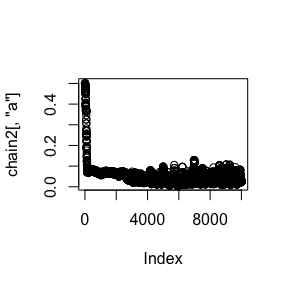We can remove a burn-in section of the chains and thin the remainder using functions burn_in and thinning:

thinned_1 <- thinning(burn_in(chain1, 4000), 100)
thinned_2 <- thinning(burn_in(chain2, 4000), 100)
plot(thinned_1[, "a"])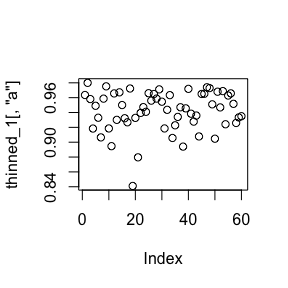plot(thinned_2[, "a"])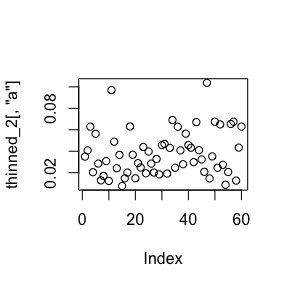hist(thinned_1[, "a"])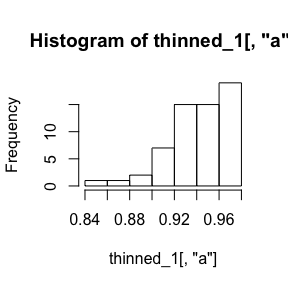hist(thinned_2[, "a"])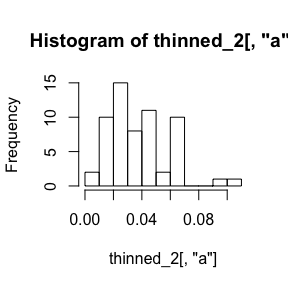We can obtain a model likelihood – capturing the likelihood of a graph topology by integrating over its parameter – using the model_likelihood function.

model_likelihood(thinned_1[, "likelihood"])
#>  -10.6736
model_likelihood(thinned_2[, "likelihood"])
#>  -9.081603

This likelihood is in log-space and computing it requires the addition of many likelihood values that can vary in absolute magnitude, a process that is potentially numerically unstable. To get a feeling of the numerical stability of this computation we can permute the likelihoods and capture the variation in results. For this we can use the model_likelihood_n function, where n refers to the number of permutations to use.

model_likelihood_n(thinned_1[, "likelihood"], 100)
#>           mean        sd
#> [1,] -10.03596 0.7536685
model_likelihood_n(thinned_2[, "likelihood"], 100)
#>           mean        sd
#> [1,] -9.429913 0.3275259

The result shows the mean log-likelhood over the permutations together with the standard deviation over permutations.

The logarithm of the Bayes factor comparing the two models can be obtained just from subtracting one log-likelihood from the other, but we can obtain it together with the variation due to the numerical algorithm using the model_bayes_factor_n function:

model_bayes_factor_n(thinned_1[, "likelihood"], thinned_2[, "likelihood"], 100)
#>           mean        sd
#> [1,] -0.552034 0.9190712

The result here shows a non-significant support (Bayes factor 1.66) for the first model compared to the second.